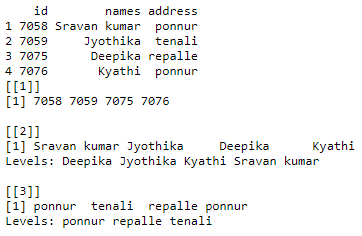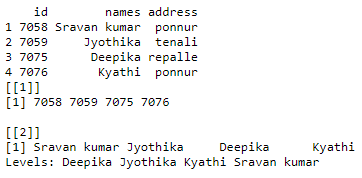# How to select multiple DataFrame columns by name in R ?

• Last Updated : 28 Apr, 2021

In this article, we will discuss how to select multiple columns from a DataFrame by name in R Programming Language. To get multiple columns we will use the list data structure. By using a list we can pass the dataframe columns separated with a comma. Then, we can get list by using list() function

Syntax:

list(dataframe_name\$column1,dataframe_name\$column2,.,dataframe_name\$column n)

Example1:

## R

 `# create a vector with student id``vector1=``c``(7058,7059,7075,7076)`` ` `# create a vector with student name``vector2=``c``(``"Sravan kumar"``,``"Jyothika"``,``          ``"Deepika"``,``"Kyathi"``)`` ` `# create a vector with student address``vector3=``c``(``"ponnur"``,``"tenali"``,``"repalle"``,``          ``"ponnur"``)`` ` `# pass these vectors to the dataframe``dataframe1=``data.frame``(id=vector1,names=vector2,``                      ``address=vector3)`` ` `print``(dataframe1)`` ` `# select multiple columns from the``# dataframe1 using list datastructure``a=``list``(dataframe1\$id,dataframe1\$names,dataframe1\$address)`` ` `print``(a)`

Output:Example 2:

## R

 `# create a vector with student id``vector1=``c``(7058,7059,7075,7076)`` ` `# create a vector with student name``vector2=``c``(``"Sravan kumar"``,``"Jyothika"``,``          ``"Deepika"``,``"Kyathi"``)`` ` `# create a vector with student address``vector3=``c``(``"ponnur"``,``"tenali"``,``"repalle"``,``"ponnur"``)`` ` `# pass these vectors to the dataframe``dataframe1=``data.frame``(id=vector1,names=vector2,``                      ``address=vector3)`` ` `print``(dataframe1)`` ` `# select multiple columns from the``# dataframe1 using list datastructure``a=``list``(dataframe1\$id,dataframe1\$names)`` ` `print``(a)`

Output:My Personal Notes arrow_drop_up